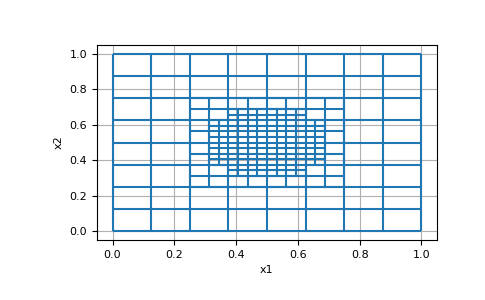# discretize.TreeMesh.refine¶

TreeMesh.refine(self, function, finalize=True)

Refine `TreeMesh` with user-defined function.

Refines the `TreeMesh` according to a user-defined function. The function is recursively called on each cell of the mesh. The user-defined function must accept an object of type `TreeCell` and must return an integer-like object denoting the desired refinement level. Instead of a function, the user may also supply an integer defining the minimum refinement level for all cells.

Parameters
function

a function defining the desired refinement level, or an integer to refine all cells to at least that level. The input argument of the function must be an instance of `TreeCell`.

finalizebool, `optional`

whether to finalize the mesh

Examples

Here, we define a QuadTree mesh with a domain width of 1 along the x and y axes. We then refine the mesh to its maximum level at locations within a distance of 0.2 of point (0.5, 0.5). The function accepts and instance of `TreeCell` and returns an integer value denoting its level of refinement.

```>>> from discretize import TreeMesh
>>> from matplotlib import pyplot
```

Define a mesh and refine it radially outward using the custom defined function

```>>> mesh = TreeMesh([32,32])
>>> def func(cell):
...     r = np.linalg.norm(cell.center-0.5)
...     return mesh.max_level if r<0.2 else mesh.max_level-2
>>> mesh.refine(func)
```
```>>> mesh.plot_grid()
>>> pyplot.show()
```